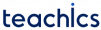# Arrays in C

An Array is a collection of variables of a similar type that are referenced by a common name. Each data item in an array is called an element. The array elements are stored in contiguous memory locations. Elements can be accessed randomly using indices(subscripts) of an array.

The pictorial representation of an array is given below.

The size of the above array is 7. The first index = 0 and the last index = 6.

### Declaring an Array in C

The syntax for declaring an array of size n in C is as follows,

`dataType arrayName[size];`

For example, an array Arr of type integer having a size of 7 can be declared as follows,

`int Arr;`

### Initializing an Array in C

Arrays in C can be initialized during declaration. For example,

`int Arr={22,32,44,51,65,71,80}`

You can also initialize an array without specifying the size like this,

`int Arr[]={22,32,44,51,65,71,80}`

Since we are initialized the array with 7 elements, the compiler will assume its size is 7.

### Accessing Array elements in C (Read and Write)

Elements of an array can be accessed by indices. Array indices start at 0 and go till size-1.

Consider the above-mentioned array Arr of size 7. The first element is Arr, the second element is Arr and the last element is Arr.

### How to take user input and save it in an array element?

Here is how you can take user input and save it as the fourth element of array Arr.

`scanf("%d", Arr);`

### Update values of array elements

You can also update the values of array elements like this. For example, you can make the value of the fourth element of the array Arr to 92 as below.

`Arr = 92; `

### Representation of Arrays in memory

In C, all arrays consist of contiguous memory locations. So, the computer only needs to track the address of the first element of an array(Base address).

Suppose the base address of the array Arr is 1000. Then, the address of Arr will be 1002. Likewise, the address of Arr will be 1004 and so on. This is because the size of int is 2 bytes.

```#include <stdio.h>

int main() {
int marks;

printf("Enter marks of 5 subjects: ");

// taking input from user and storing it in array mark
for(int i = 0; i < 5; ++i) {
scanf("%d", &marks[i]);
}

printf("The marks you entered are: ");

// printing elements
for(int i = 0; i < 5; ++i) {
printf("%d\n", marks[i]);
}
return 0;
}```
##### Output
```Enter marks of 5 subjects: 23
45
38
29
48
The marks you entered are: 23
45
38
29
48```

### How to insert and delete elements at the desired position in an array?

Inserting or deleting an element at the of an array can be easily done. If we need to insert or remove an element in the middle of an array, half of the items must be shifted to accommodate the new element while maintaining the order of the other elements.

In the next tutorial let’s learn How to insert and delete elements at the desired position in an array?

### Multi-dimensional arrays

The arrays we learned in this tutorial are called one-dimensional arrays. Follow the tutorial with examples to learn about multi-dimensional arrays in C.

Subscribe
Notify of